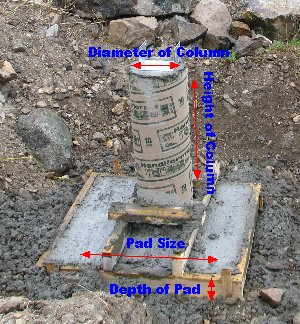Spike's Calculators

# Columns with Pads Concrete Volume CalculatorCalculate the amount of concrete needed for a column and pad using feet and inches as measurements.

Columns need to be supported by a footing. In some cases, these can be the same footings that support your foundation wall, but in most cases, they need to have a separate footing, also known as a pad. For round columns, these pads usually are square.

To calculate the volume of concrete for a column, you need the diameter and height. And for a square pad, the length of one side and the thickness (depth) of the pad.# Number Columns and Pads #
Column Diameter in
Column Height ft in
Depth in

Lineal Foot Length of Tube lin/ft
Cubic Feet ft ³
Cubic Yards yd ³
Cubic Metres

#### Calculation

1. enter the number of columns and pads
2. the diameter of the column in inches
3. the height of the column in feet and inches
4. length of one side of the pad in feet and inches
5. depth of the pads in inches

#### Results

1. lineal feet of forming tube for the columns (rounded up)
2. forming material for the pads, lineal feet (rounded up)
3. amount of concrete needed in cubic feet
4. concrete needed in cubic yards
5. concrete needed in cubic metres

##### Formula
for the column;
`V = π * D² ÷ 4 * h * n`
`V = w² * d * n`

```where V = volume
π = 3.14159...,
D = diameter
h = height of the column
n = number of pads and columns```

##### Example calculation;
```column diameter = 10 inches
column height = 4 feet -  8 inches
pad size = 28 inches  x 28 inches
thickness of pad = 10 inches

Since it is easier to calculate using the same units, convert the 4 feet - 8 inches into inches by multiplying the feet amount by 12 ( 12 inches in one foot);
`4 * 12 + 8  = 56`
`4 feet - 8 inches =  56 inches`

Using the formula for the columns from above;
`3.14159 * 10² ÷ 4 * 56 * 4 `
`3.14159 * 100 ÷ 4 * 56 * 4 = 17592.92 rounded to two decimals`
`The volume of the  four columns is 17592.92 cubic inches`

Using the formula for the pads from above;
`28² * 10 * 4`
`784 * 10 * 4 =  31360`
`The volume of the  four pads is 31360 cubic inches`

Adding both results and converting the cubic inches to cubic feet by dividing the cubic inch amount by 1728 (there are 1728 cubic inches in one cubic foot)
`(17592.92 + 31360) ÷ 1728`
`48,952.92 ÷ 1728 = 28.3 rounded to one decimal`
`You will need 28.3 cubic feet of concrete to pour these four columns and pads.`

### When Ordering Concrete

This concrete calculator will help you in estimating the amount of concrete needed for your project. The amount given as needed, does not include any waste. It is recommended, depending on the job you are doing, to add anywhere from an extra 4% to 10% to your concrete order to make sure you have enough concrete to finish the job. Ask when ordering the concrete. They will be able to give you a good idea of what is needed.